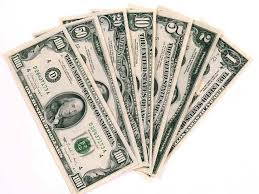## Pages

### COST OF USING ELECTRICAL ENERGY BASIC INFORMATION

POWER CONSUMPTION COMPUTATION TUTORIALSA watt-hour meter is always connected into some part of the service-entrance equipment to record the amount of energy used. Billing by the utility is generally done on a monthly basis.

The meter might be mounted on the side of the house or on a pedestal somewhere on the premise on the lot line. The utility makes this decision.

The residence discussed in this text has one meter, mounted on the back of the house near the sliding doors of the Master Bedroom. The kilowatt (kW) is a convenient unit of electrical power.

One thousand watts (w) is equal to one kilowatt. The watt-hour meter measures and records both wattage and time.

For residential metering, most utilities have rate schedules based on “cents per kilowatt-hour.” Stated another way: How much wattage is being used and for how long?

Burning a 100-watt light bulb for 10 hours is the same as using a 1000-watt electric heater for 1 hour. Both equal 1 kilowatt-hour.

kWh = WATTS X HOURS/1000 = 100X10/1000 = 1 kWh

In these examples, if the electric rate is \$0.08 cents per kilowatt-hour, the cost to operate the 100-watt light bulb for 10 hours and the cost to operate the electric heater for 1 hour are the same—\$0.08. Both loads use 1 kilowatt-hour of electricity.EXAMPLE
Find the cost of operating a color television set for 8 hours. The label on the back of the television set indicates 175 watts. The electric rate is \$0.10494 per kilowatt-hour.

Cost = 175 x 8 x \$0.10494/ 1000
=  \$ 0. 146 9 (approx. 15¢)